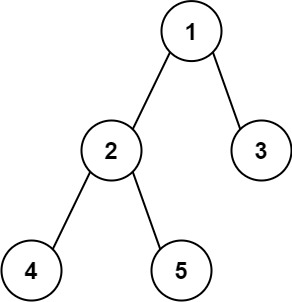0543. Diameter of Binary Tree

# 543. Diameter of Binary Tree#

## 题目 #

Given the `root` of a binary tree, return the length of the diameter of the tree.

The diameter of a binary tree is the length of the longest path between any two nodes in a tree. This path may or may not pass through the `root`.

The length of a path between two nodes is represented by the number of edges between them.

Example 1:``````Input: root = [1,2,3,4,5]
Output: 3
Explanation: 3 is the length of the path [4,2,1,3] or [5,2,1,3].

``````

Example 2:

``````Input: root = [1,2]
Output: 1

``````

Constraints:

• The number of nodes in the tree is in the range `[1, 104]`.
• `100 <= Node.val <= 100`

## 解题思路 #

• 简单题。遍历每个节点的左子树和右子树，累加从左子树到右子树的最大长度。遍历每个节点时，动态更新这个最大长度即可。

## 代码 #

``````package leetcode

import (
"github.com/halfrost/leetcode-go/structures"
)

// TreeNode define
type TreeNode = structures.TreeNode

/**
* Definition for a binary tree node.
* type TreeNode struct {
*     Val int
*     Left *TreeNode
*     Right *TreeNode
* }
*/

func diameterOfBinaryTree(root *TreeNode) int {
result := 0
checkDiameter(root, &result)
return result
}

func checkDiameter(root *TreeNode, result *int) int {
if root == nil {
return 0
}
left := checkDiameter(root.Left, result)
right := checkDiameter(root.Right, result)
*result = max(*result, left+right)
return max(left, right) + 1
}

func max(a int, b int) int {
if a > b {
return a
}
return b
}
``````Apr 8, 2023Edit this page